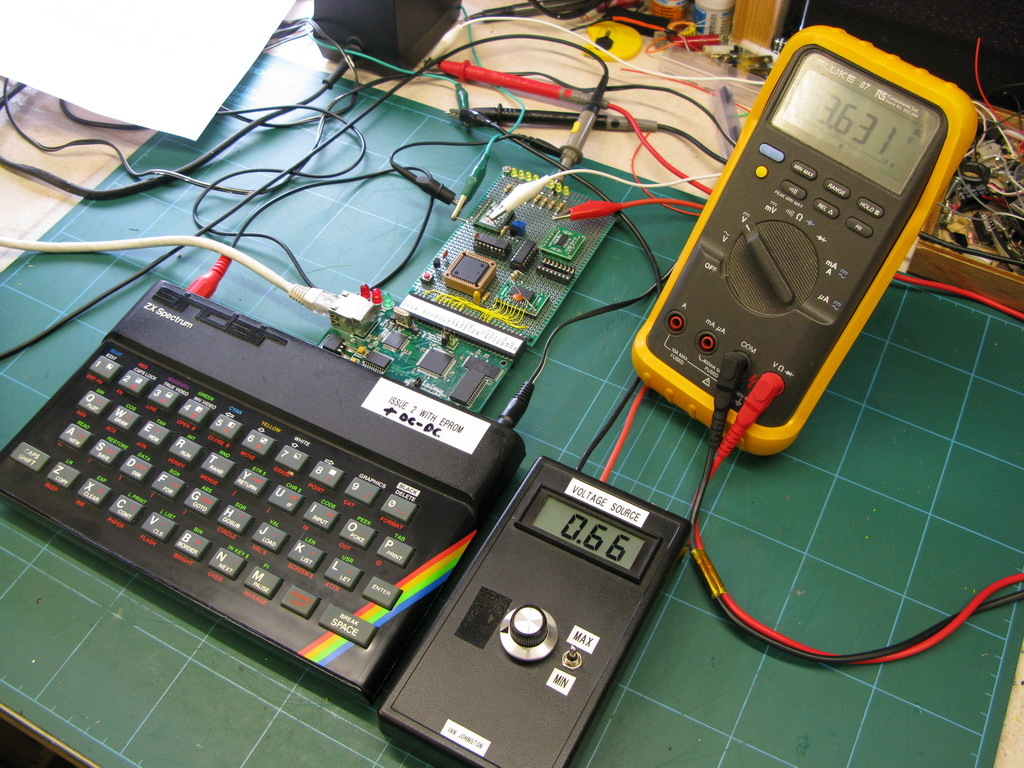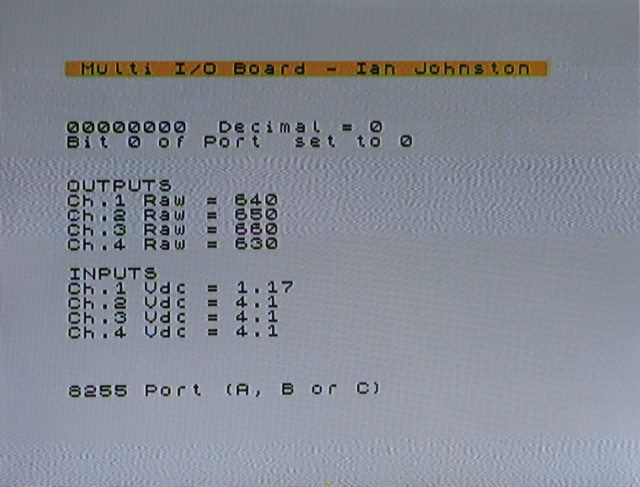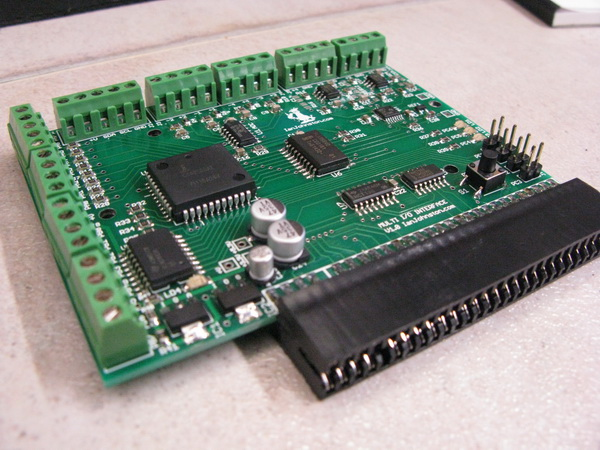• 0 Vote(s) - 0 Average
• 1
• 2
• 3
• 4
• 5
 Show off your creativity!nitrofuranoSenior MemberPosts: 366 Threads: 186 Joined: Sep 2011 Reputation: 0 11-03-2011, 01:55 PM btw, i updated http://nitrofurano.altervista.org/retroc...xspectrum/ again, with some stuff i posted here in the forumslenkarSenior MemberPosts: 282 Threads: 48 Joined: Feb 2011 Reputation: 0 11-03-2011, 06:33 PM nice screenshots of your speccy stuff (text and lines) (but the flashing on the main page is distressing)britlionPosting FreakPosts: 805 Threads: 135 Joined: Apr 2009 Reputation: 5 11-03-2011, 10:25 PM I have to agree... I didn't get further than the main page. Big flashing screens aren't something I can deal with without getting a headachenitrofuranoSenior MemberPosts: 366 Threads: 186 Joined: Sep 2011 Reputation: 0 11-03-2011, 10:45 PM i were expecting for such reaction!- but btw, M.I.A.'s myspace webpage were far worse than that!nitrofuranoSenior MemberPosts: 366 Threads: 186 Joined: Sep 2011 Reputation: 0 11-03-2011, 10:50 PM removed the flashingIanJJunior MemberPosts: 4 Threads: 0 Joined: Aug 2014 Reputation: 0 09-03-2014, 04:25 PM Hi all, For fun i've built a wee interface for my own ZX Spectrum which gives the Speccy:- 4off 16bit analogue inputs (configurable from 12-bit to 18bit) 4off 16bit analogue outputs 24off digital I/O I2C bus And here it is running off the back of a Spectranet card.Screenshot of test screen:-My first ZXBC compiled prog, here's the source code (work in progress). I don't mind it going public, it's only for fun. Couldn't have done it without ZXBC............ Ian. Code:```' ZX Spectrum Multi IO board ' By Ian Johnston 24/08/2014 ' 82C55 Port A = 8off Outputs ' 82C55 Port B = 8off Inputs ' 82C55 Port C = 4off Inputs (lower) '                4off Outputs (upper) ' ' DAC8574IPW 4ch DAC IC, 16bit ' MCP3424 4ch ADC IC, 12-18bit running in 12bit mode ' ' This code is designed to be compiled to TAP using ZX Basic compiler (www.boriel.com)     #include      ' Vars     DIM DacSend(3) AS INTEGER     DIM DacCh(4) AS UInteger     DIM digA AS UInteger: LET digA=0     DIM digB AS UInteger: LET digB=0     DIM A AS UByte: LET A=0     DIM B AS UByte: LET B=0     DIM conv AS UByte: LET conv=0     DIM digout AS UInteger: LET digout=0     DIM AdcReceive(2) AS UByte                ' 16bit max with 2 vars     DIM AdcCh AS Float                        ' 16bit max 0-65535 (this was UInteger but needs to be float for VoltCh)     DIM Adcconfig AS UInteger     DIM Adcval(4) AS Float     DIM Voltch(4) AS Float     DIM Adcchconfig(4) AS UInteger: LET Adcchconfig(1)=184: LET Adcchconfig(2)=216: LET Adcchconfig(3)=248: LET Adcchconfig(4)=152     DIM adc AS UByte=0     DIM dac AS UByte=0     DIM i AS UByte ''''''''''''''''''''''''''''''''''''''''''''''''''''''''''''''''''''''''''''''''''''''''''''''''''''''''''''''''' ' SETUP     ' 82C55 ports     OUT 59,131     ' temporary settings - all outputs off port A     OUT 11,0     GOSUB turnoffbit     PRINT PAPER 6;AT 0,0;" Multi I/O Board - Ian Johnston "     PRINT AT 1,0;"                                "     GOSUB pc9564init                        ' Initialize I2C controller IC ''''''''''''''''''''''''''''''''''''''''''''''''''''''''''''''''''''''''''''''''''''''''''''''''''''''''''''''''' ' MAIN LOOP mainloop:     IF INKEY\$ = "d" THEN GOSUB diginputfromuser: END IF     ' DAC     LET dac=dac+1     IF dac=5 THEN LET dac=1: END IF     GOSUB dac8574                            ' DAC IC     ' ADC     LET adc=adc+1     IF adc=5 THEN LET adc=1: END IF     GOSUB mcp3424chchange                    ' Config ADC channel     GOSUB mcp3424                            ' ADC IC     ' Testing only     FOR i=1 TO 4     LET DacCh(i)=DacCh(i)+10      '(i*2)     IF DacCh(i)>=65535 THEN LET DacCh(i)=1: END IF     NEXT i     GOTO mainloop ''''''''''''''''''''''''''''''''''''''''''''''''''''''''''''''''''''''''''''''''''''''''''''''''''''''''''''''''' ' Digital outputs - Input from user diginputfromuser:     PRINT AT 22,0;"8255 Port (A, B or C)"     port\$=INPUT (1)     PRINT AT 22,0;"Bit (0 to 7, or 8 for all bits)"     b\$=INPUT (1): LET bit = VAL(b\$)     PRINT AT 22,0;"On/Off (1 or 0)"     f\$=INPUT (1): LET onoff = VAL(f\$)     PRINT AT 22,0;"                               "     IF onoff = 0 THEN BEEP 0.02,20: GOSUB turnoffbit: END IF     IF onoff = 1 THEN BEEP 0.02,20: GOSUB turnonbit: END IF     RETURN ' Turn off bit turnoffbit:     IF bit=0 THEN LET digB=254: END IF     IF bit=1 THEN LET digB=253: END IF     IF bit=2 THEN LET digB=251: END IF     IF bit=3 THEN LET digB=247: END IF     IF bit=4 THEN LET digB=239: END IF     IF bit=5 THEN LET digB=223: END IF     IF bit=6 THEN LET digB=191: END IF     IF bit=7 THEN LET digB=127: END IF     IF bit=8 THEN LET digB=0: END IF     ' Bitwise AND and retain copy of current outputs in digA     LET digout = digA bAND digB: LET digA = digout     IF port\$="a" THEN OUT 11,digout: BEEP 0.05,10: END IF     IF port\$="b" THEN OUT 27,digout: BEEP 0.05,10: END IF     IF port\$="c" THEN OUT 43,digout: BEEP 0.05,10: END IF     LET conv=1: GOSUB binaryconv     PRINT AT 4,0;s\$;"  Decimal = ";digout;"  "     PRINT AT 5,0;"Bit ";bit;" of Port ";port\$;" set to ";onoff     RETURN ' Turn on bit turnonbit:     IF bit=0 THEN LET digB=1: END IF     IF bit=1 THEN LET digB=2: END IF     IF bit=2 THEN LET digB=4: END IF     IF bit=3 THEN LET digB=8: END IF     IF bit=4 THEN LET digB=16: END IF     IF bit=5 THEN LET digB=32: END IF     IF bit=6 THEN LET digB=64: END IF     IF bit=7 THEN LET digB=128: END IF     IF bit=8 THEN LET digB=255: END IF     ' Bitwise OR and retain copy of current outputs in digA     LET digout = digA bOR digB: LET digA = digout     IF port\$="a" THEN OUT 11,digout: BEEP 0.05,10: END IF     IF port\$="b" THEN OUT 27,digout: BEEP 0.05,10: END IF     IF port\$="c" THEN OUT 43,digout: BEEP 0.05,10: END IF     LET conv=1: GOSUB binaryconv     PRINT AT 4,0;s\$;"  Decimal = ";digout;"  "     PRINT AT 5,0;"Bit ";bit;" of Port ";port\$;" set to ";onoff     RETURN ' Binary conversion, s\$ is the output binaryconv:     LET s\$=""     IF conv=0 THEN LET n=A: END IF     IF conv=1 THEN LET n=digout: END IF     LET s\$ = ""     binloop:     LET aa = n * 0.5     IF aa = 0 THEN GOTO addzeros: END IF     IF aa > INT aa THEN LET s\$ = "1" + s\$: LET n = ((n - 1)/2): GOTO binloop: END IF     IF aa <= INT aa THEN LET s\$ = "0" + s\$: LET n = aa: GOTO binloop: END IF     ' IF conv=1 THEN PRINT AT 8,0;s\$: END IF addzeros:     IF LEN s\$ = 7 THEN LET s\$ = "0" + s\$: END IF     IF LEN s\$ = 6 THEN LET s\$ = "00" + s\$: END IF     IF LEN s\$ = 5 THEN LET s\$ = "000" + s\$: END IF     IF LEN s\$ = 4 THEN LET s\$ = "0000" + s\$: END IF     IF LEN s\$ = 3 THEN LET s\$ = "00000" + s\$: END IF     IF LEN s\$ = 2 THEN LET s\$ = "000000" + s\$: END IF     IF LEN s\$ = 1 THEN LET s\$ = "0000000" + s\$: END IF     IF LEN s\$ = 0 THEN LET s\$ = "00000000": END IF     ' PRINT AT 21,0;"           "     ' IF conv=1 THEN PRINT AT 9,0;s\$: END IF     RETURN ''''''''''''''''''''''''''''''''''''''''''''''''''''''''''''''''''''''''''''''''''''''''''''''''''''''''''''''''' ' I2C PCS9564 ' Initialize pc9564init:     OUT 43,0                                ' Set PC7 low then high to reset PCA9564 IC     PAUSE 5     OUT 43,128     PAUSE 5     OUT 13,127                                ' Timeout function (MSB) enabled = 255, disabled = 127     OUT 45,100                                ' PCS9564 I2C address = 0x64 = 100     OUT 61,68                                ' Clock freq serial = 0x44 = 68 = 88kHz     RETURN ''''''''''''''''''''''''''''''''''''''''''''''''''''''''''''''''''''''''''''''''''''''''''''''''''''''''''''''''' ' DAC IC = DAC8574 ' Master Tx mode dac8574:     OUT 61,228                                ' Start command E4 = 228     ' Setup Slave device (Tx mode)     OUT 29,152                                ' Load Slave addr + R/W bit = 0 into I2CDAT (DAC8574IPW 0x4c = 76 = 1001100) + R/W bit= 10011000 = 152     OUT 61,196                                ' Reset SI and STA bits in I2CCON     ' Send 1-byte at a time. Channel codes: 32,34,36,38 then MSB,LSB     ' Build LSB (max int 65535)     PRINT AT 8,0;"OUTPUTS"     PRINT AT 8+dac,0;"Ch.";dac;" Raw = ";DacCh(dac);"       "     LET A=DacCh(dac): LET B=255     LET A = A bAND B     LET l=A     ' Build MSB     LET A=DacCh(dac)/256     LET m=INT A     ' Build data array ready for sending to the DAC: CHANNEL, MSB, LSB     IF dac=1 THEN LET DacSend(1)=32: LET DacSend(2)=m: LET DacSend(3)=l: END IF     IF dac=2 THEN LET DacSend(1)=34: LET DacSend(2)=m: LET DacSend(3)=l: END IF     IF dac=3 THEN LET DacSend(1)=36: LET DacSend(2)=m: LET DacSend(3)=l: END IF     IF dac=4 THEN LET DacSend(1)=38: LET DacSend(2)=m: LET DacSend(3)=l: END IF     ' Send data     FOR i = 1 TO 3     OUT 29, DacSend(i)                        ' Load data into I2CDAT, transfer data to DAC     FOR delay=1 to 4: NEXT delay     OUT 61,196                                ' Reset SI and STA bits in I2CCON     FOR delay=1 to 4: NEXT delay     'PRINT AT 6,0;dac;" ";DacSend(1)     NEXT i     OUT 61,212                                ' Generate stop command, write I2CCON with 0xD4 (212)     RETURN ''''''''''''''''''''''''''''''''''''''''''''''''''''''''''''''''''''''''''''''''''''''''''''''''''''''''''''''''' ' ADC IC ADC = MCP3424 ' Master Rx mode mcp3424:     OUT 61,228                                ' Start command E4 = 228     ' Setup Slave device - receive data from device     OUT 29,211                                ' Load Slave addr + R/W bit = 1(read) into I2CDAT (MCP3424 0x69 = 105 = 1101001) + R/W bit= 11010011 = 211     OUT 61,68                                ' Reset SI and STA bits in I2CCON (0xC4 = 196), or 68 if AA is set to OFF     FOR delay=1 to 4: NEXT delay     ' Receive data     FOR i = 1 TO 2     OUT 61,196                                ' Reset SI and STA bits in I2CCON     FOR delay=1 to 4: NEXT delay     LET AdcReceive(i)=IN 29    ' Read byte out     NEXT i     OUT 61,68                                ' Reset SI and STA bits in I2CCON     FOR delay=1 to 4: NEXT delay     OUT 61,212                                ' Generate stop command, write I2CCON with 0xD4 (212)     Let MSB = AdcReceive(1) * 256            ' <<     Let LSB = AdcReceive(2) bAND 255        ' &     Let AdcCh = MSB bOR LSB                    ' |     LET Adcval(adc) = AdcCh    ' Load 4 channel values on at a time     ' PRINT AT 11,0;"Ch.1 Inp = ";AdcCh1;" MSB=";AdcReceive(1);" LSB=";AdcReceive(2);"   "     LET Voltch(adc) = Adcval(adc) / 15968     LET Voltch(adc)=Voltch(adc)*100: LET Voltch(adc)=INT(Voltch(adc)): LET Voltch(adc)=Voltch(adc)/100        ' Round down to 2 DP's          PRINT AT 14,0;"INPUTS"     PRINT AT (14+adc),0;"Ch.";adc;" Vdc = ";Voltch(adc);"  "      ';"  ";Adcval(adc);"  ";AdcCh;"  ";Adcchconfig(adc)     RETURN ''''''''''''''''''''''''''''''''''''''''''''''''''''''''''''''''''''''''''''''''''''''''''''''''''''''''''''''''' ' ADC IC ADC = MCP3424 ' Master Tx mode (to set up channel config only) mcp3424chchange:     OUT 61,228                                ' Start command E4 = 228     ' Setup Slave device (Tx mode)     OUT 29,210                                ' Load Slave addr + R/W bit = 0 into I2CDAT (MCP3424 0x69 = 105 = 1101001) + R/W bit= 11010010 = 210     OUT 61,196                                ' Reset SI and STA bits in I2CCON     ' Send data     PAUSE 1                                    ' Important pause otherwise the data does not go out on the I2C bus properly.     OUT 29, Adcchconfig(adc)                ' configuration byte = initiate,Ch.n,continuous,16-bit,x1Gain     PAUSE 1     OUT 61,196                                ' Reset SI and STA bits in I2CCON     PAUSE 1     OUT 61,212                                ' Generate stop command, write I2CCON with 0xD4 (212)     ' 3910 FOR delay=1 to 4: NEXT delay     RETURN```borielAdministratorPosts: 1,664 Threads: 54 Joined: Aug 2019 Reputation: 9 09-03-2014, 05:05 PM OMG!!!!! This is absolutely awesome!! :o :o :o I want that!nitrofuranoSenior MemberPosts: 366 Threads: 186 Joined: Sep 2011 Reputation: 0 09-07-2014, 09:49 PM i indexed it on the wiki right now! \o/ - http://www.boriel.com/wiki/en/index.php ... .2FO_BoardIanJJunior MemberPosts: 4 Threads: 0 Joined: Aug 2014 Reputation: 0 10-10-2014, 06:55 PM Hi all, Finished project:- http://www.ianjohnston.com/index.php/pr ... -interface Thanks to Boriel ZX Basic........without it my project would never have happened! Ian.nitrofuranoSenior MemberPosts: 366 Threads: 186 Joined: Sep 2011 Reputation: 0 10-11-2014, 12:06 PM excellent!« Next Oldest | Next Newest »

Forum Jump:

Users browsing this thread: 1 Guest(s)